Eric Chapdelaine
Student at Northeastern University Studying Computer Science.
Notes
Projects
Articles
Resume

Email
GitHub

# Module 2 - Statement Representation

## Circuits and Logic

• OR
• XOR
• XNOR
• NANDS Is the sum and c is the carry. Uses xnor for the sum because 0 xnor 0 = 0, 1 xnor 0 = 1, and 1 xnor 1 = 0 (where the carry is).

What if you want a third input? You have to expand the Half Adder## Boolean Algebra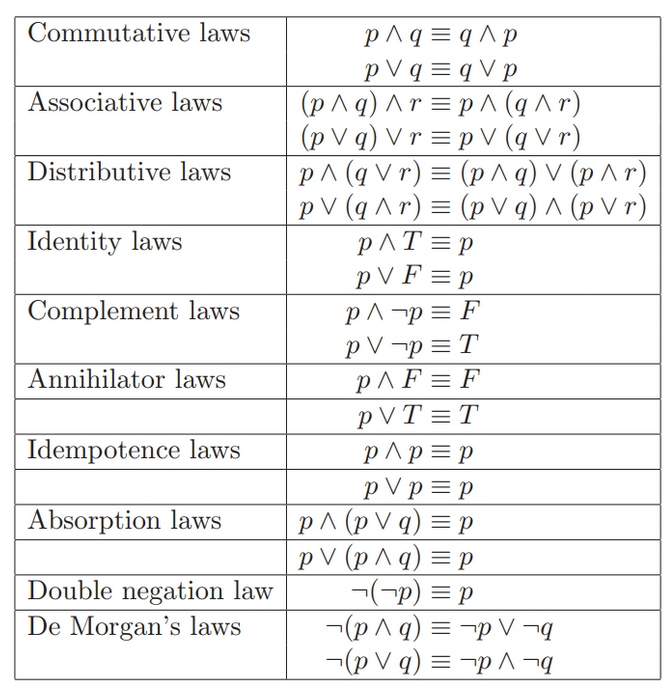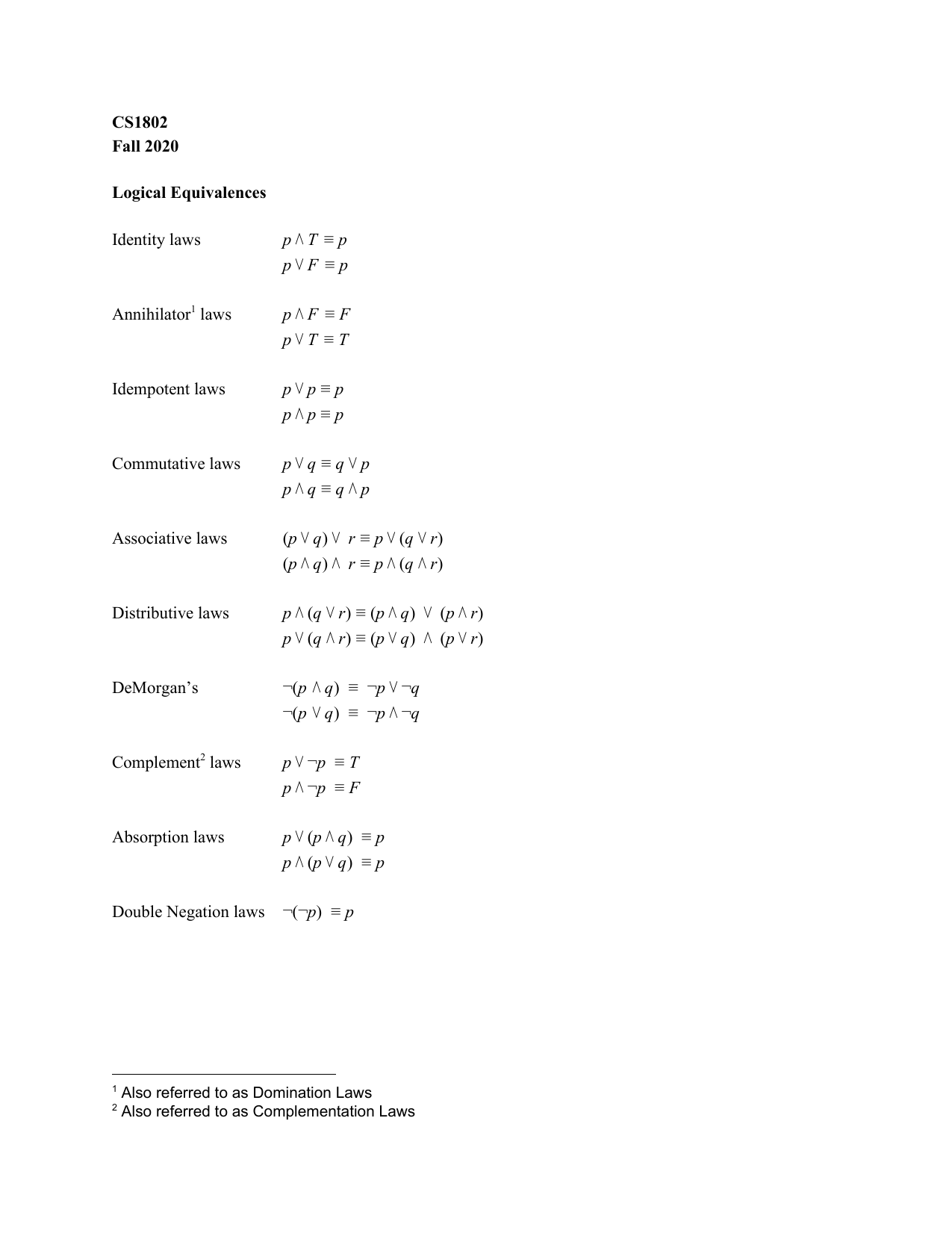Boolean Equivalence

$(A \wedge \neg B) \vee (A \vee B) \equiv A$

Distributive Law: $((A \wedge \neg B) \vee A) \wedge ((A \wedge \neg B) \vee B)$

Commutative Law: $(A \vee (A \wedge \neg B)) \wedge ((A \vee B) \wedge (B \vee \neg B))$

Absorption Law and Known Always True: $A \wedge (A \vee B)$

Absorption: $A$

## Implications, Inverses and Contrapositives

### Conditionals/Implications

• If p then q

Example:

p: Ben left the house after 8am

q: Ben arrived at work after 9am

If Ben left after 8am, then Ben arrived after 9pm

$p \to q$ (we use double arrow notation $\implies$)

“If p then q”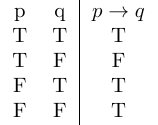What if we flipped the truth table?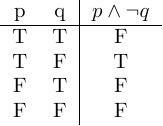This proves that

$\neg (p \to q) \equiv p \wedge \neg q$ $p \implies q \equiv \neg(p \land \neg q) \equiv \neg p \lor q$

### Contrapositives

$p \to q \equiv \neg q \to \neg p$

If Ben arrived before 9, he must’ve left before 8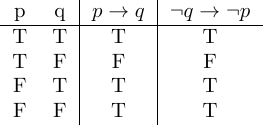### Converse of $p \to q$ is $q \to p$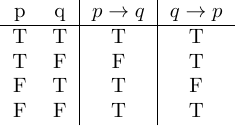If Ben arrives after 9, then Ben left after 8

### Inverse $\neg p \to \neg q$

(Contrapositive of the Converse)

## Existential Qualifiers

Quantities

• None
• Some
• All

It rained every first day of the month last string (March, April, May)

On every first day of the month, it rained

On every x, it rained

$p(x)$= it rained on $x$ →on every $x$, $p(x)$

for all first days of the month, it rained

• For all $x$, it rained
• for all, $x$, $p(x)$

Denotated as:

$\forall x, p(x)$

$x$ is the first day of the month

$x \in$ first days of the month

$\forall$ - Universial Quantifiers

• On every
• For all
• For every
• For each
• All of

Example

$Q(x)$ : $x$ has green leaves, $x$ is a tree

$\forall x, Q(x)$ : Every tree has green leaves

• NOT True

Some trees have red leaves → There is a tree with red leaves (only have to prove one)

$R(x)$ : $x$ has red leaves

$\exists x, R(x)$

There exists a tree with red leaves

Example:

$\forall x, R(x)$ - All trees have red leaves

Negate: Exhibit a tree with leaves that aren’t real. Some trees don’t have red leaves

Therefore:

$\forall x, R(x)$

Negate: $\exists x, \neg R(x)$

Example: There are some trees that have purple leaves.

Every tree doesn’t have purple leaves

$\exists x, purple(x)$

Negate: $\forall x, \neg purple(x)$

Summary:

$\neg \forall x, p(x) \equiv \exists x, \neg p(x)$

$\neg \exists x, R(x) \to \forall x, \neg R(x)$

None: $\forall x \neg p(x)$

All : $\forall$

Some: $\exists$

# Module 3 - Collection Representation

## Lesson 1 - Set Representation

Set of locations that Northeastern has a campus in (each one of them is called an element):

• London
• Portland
• Boston

NOTE: Sets cannot have duplicates

### Notation

NU_CAMPUS = {London, Portland, …, Boston}

$\in$ - an element of

Ex. London $\in$ NU_CAMPUS

### Infinite Sets

In order to represent infinite sets (ex. multiples of 5), we need to use set builder notation

Multiple of 5:

 { x x = 5 * n, n ≤ 1}

This example isn’t too clear because the ncan be any number like 1.3, not just natrual numbers.

### Numbers

• Natrual - $\N$
• Integers - $\Z$
• Rationals - $\mathbb{Q}$
• Real - $\R$

Therefore the multiple of 5 example should look like this:

 { x x = 5 * n, n $\in \N$} or { 5n n $\in \N$}

The first one is perferred because it represents computer programming languages

### General Notation

 { x the rules governing membership}

## Lesson 2: Subsets, The Empty Set and The Power Set

Recall:

NU_CAMPUS = {London, Portland, …, Boston}

NU_US_CAMPUSES = {Boston, Seattle, …}

Subsets - $\subseteq$

NU_US_CAMPUSES $\subseteq$ NU_CAMPUS

Another example: Fives = { x | x = 5 * n, n $\in \N$}

 Tens = { x x = 10 * n, n $\in \N$}

Tens $\subseteq$ Fives

### General Notation

If and only if - $\iff$

Ex:

A $\subseteq$ B $\iff$ $\forall$ x $\in$ A, x $\in$ B

If not in a subset:

A $\nsubseteq$ B $\iff$$\exists x \in A, x \notin B Proper Subset - \subset C \subset D \iff$$\forall$ x $\in$ C, x $\in$ D and $\exists$ y $\in$ D, y $\in$ C

E $\subseteq$ F and F $\subseteq$ E $\iff$E $\equiv$ F (very helpful for proofs)

### The Empty Set

• Represented with {} or $\emptyset$
• Is a subset of every other set

### The Power Set

• The power set is a set of all possible subsets of any given set

Example:

IC = {chocolate, strawberry, vanilla}

$\wp (IC)$ = { {}, {chocolate, strawberry, vanilla}, {chocolate}, {strawberry}, {vanilla}, {chocolate, strawberry}, …}

Every set is a subset of itself

How many elements are in $\wp (IC)$?

• IC - 3 elements
• $\wp (IC)$ - 8 elements

Notice that $8 = 2^{3}$

We can make this into a general form:

• A - n elements
• $\wp (A)$ - $2^{n}$ elements

## Lesson 3: Operations on Sets

• Complement of a set
• Union
• Intersection
• Difference
• Cartesian Product

Favorite Green Vegetables (FGV) - there is an implied context. It is Ben’s favorite things in the universe green vegetables

Universe - $\mho$

FGV = {cucumber, peas, lettuce, broccoli}

Venn Diagram Representations of Sets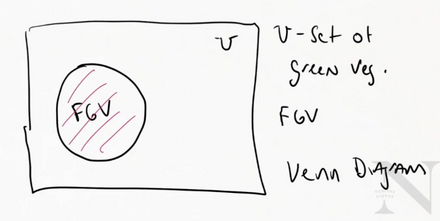### Complement

Complement of FGV

• All green vegetables not in that set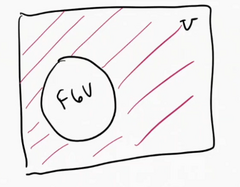The complement of a set A, is written $\bar{A}$

$\bar{A}$ is the set of all elements in a universe that aren’t in A

 Formation Definition: $\bar{A} = {x x \notin A, x \in \mho}$

NOTE: The $x \in \mho$ is optional and most often left out

FGV = {cucumber, peas, lettuce, broccoli}

MGV = {cucumber, lettuce, asparagus}

### Union

FGV $\cup$ MGV = {cucumber, lettuce, asparagus}

$A \cup B = B \cup A$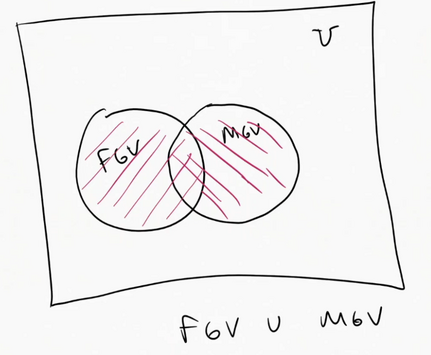The union of a set A with a set B written, $A \cup B$, is th set of all elements in A and all elements in B

Formal Definition:

 $A \cup B = {x x\in A \vee x \in B}$

### Intersection

All of the elements in common

$FGV \cap MGV = {cucumber, lettuce}$

$A \cap B = B \cap A$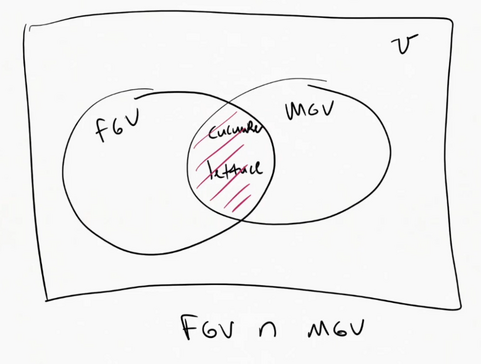The intersection of a set A with a set B is the set of all elements that are in both A and B

Formal Definition:

 $A \cap B = {x x \in A \wedge x \in B }$

Unions and intersections of more than two sets:

 $A \cup B \cup C = {x x \in A \vee x \in B \vee x \in C }$
 $A \cap B \cap C = {x x \in A \wedge x \in B \wedge x \in C }$

Formal Explanation:

$\bigcup\limits_{i=1}^{n} A_{i} = A_{1} \cup A_{2} \cup A_{3} … \cup A_{n}$

$\bigcap\limits_{i=1}^{n} A_{i} = A_{1} \cap A {2} \cap A{3} … \cap A_{n}$

### Difference

What’s in one set and not the other

• Ordered operation! $\therefore A - B \neq B - A$

Recall:

FGV = {cucumber, peas, lettuce, broccoli}

MGV = {cucumber, lettuce, asparagus}

$FGV - MGV = { peas,brocolli}$

Sometimes it’s written $FGV / MGV$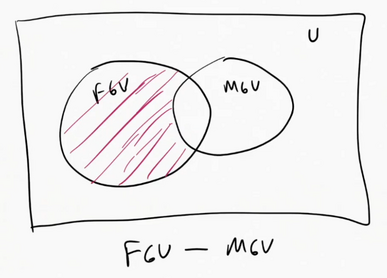The difference between set A and B, written $A-B$, is the set of all elements in A that not in B

Formal Definition:

 $A - B = {x x\in A \wedge x \notin B }$

### Cartesian Product

All possible pairs

Recall the Cartesian plane which represents all possible pairs of x and y positions

$GV = {cucumber, peas}$

$berry = {blue, straw, black }$

$GV \times berry = {(cucumber, blue), (cucumber, straw), (cucumber, black), (peas, blue), (peas, straw), (peas, black)}$

Parenthesis mean pair

Definition:

The Cartesian product of set A with set B, written $A \times B$, is the set of all possible pairs from A with the elements in B.

 $A \times B = {(x,y) x \in A \wedge y \in B}$

This is useful for pairing things in databases

### Symmetric Difference

Everything but the intersection

$A \vartriangle B = (A - B) \cup (B -A)$

## Lesson 5 - Binary Strings

Consider the universe:

$u = [vegtables, fruits, grains, protein, dairy]$

Length 5

If we have the set:

$s = {vegtables, protein}$

This set corresponds to the 1st and 4th elements in the given universe so we can denote it as:

$10010$

It’s 5 bits because the universe is length 5. Notice how only the 1st and 4th digit (from the left) is 1.

## Lesson 6 - Writing Proofs

Proof - A mathematical reason that something is true

• Arguing something

Prove that $A - B \equiv A \cap \bar{B}$

### Belief Phase

• Use the Venn diagrams to convince yourself that the statement is true

Explain the statements to their formal definitions:

 $A - B = {x x \in A \wedge x \notin B }$
 $A \cap \bar{B} = {y y \in A \wedge y \in \bar{B}}$

### Prove Idea

A rough skeleton of the formal proof. This is called a direct proof.

• How do we show set equivalence?

Set Equivalence Laws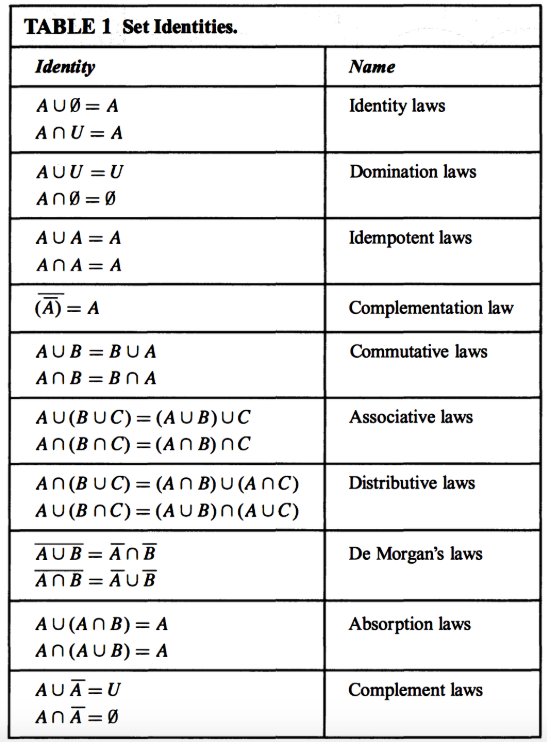In order to prove this, we need to first prove this:

$A - B \subseteq A \cap \bar{B}$ and

$A \cap \bar{B} \subseteq A - B$

$x \in A - B \implies x \in A \wedge x \notin B \implies x \in A \wedge x \in \bar{B}$

$x \in A \cap \bar{B} \implies x \in A \wedge x \in \bar{B} \implies x \in A \wedge x \notin B$

### The Actual Proof

THM: A - B \equiv A \cap \bar{B}
Proof:
To show that the set A - B is equivalent to the set A \cap \bar{B} we will
show that A - B \subseteq A \cap \bar{B} and A \cap \bar{B} \subseteq A - B.
First we will show A - B \subseteq A \cap \bar{B}. Consider the definition
of A - B, A - B = \{x|x \in A \wedge x \notin B \} since x \in A \wedge x \notin
B, we know htat x \in A \wedge x \in \bar{B} by the definition of set complement
so x \in A \cap \bar{B}. Now to show A \cap \bar{B} \subseteq A - B we again
consider the definition A \cap \bar{B} which is the set of all x, x \in A
\wedge x \in \cap{B} but since x \in \bar{B} is the same as x \notin B by
definition, we know x \in A \wege x \notin B which is the same as x \in A - B.
So when x \in A \cap \bar{B} \implies x \in A - B. So we have A - B \subseteq A
\wedge \bar{B} and A \wedge \bar{B} \subseteq A - B so A - B \equiv A \wedge
\bar{B}. \boxtimes


You end formal proofs with either $\boxtimes$ or $Q.E.D$

## Summary

2346

### Sets

Sets are collections of unique objects, meaning there are no duplicates. We can write them either in list notation or set builder notation.

$S = {0,1,2,3}$

$x \in A$: x in an element of A

$A \equiv B$: A is equivalent to B if both sets have the exact same elements

$A \subseteq B$: A is a subset of B if all elements in A are also in B.

$A \subset B$: A is a proper subset of B if A is a subset of B but A is not equivalent to B.

### Special Sets

We learned of a number of special sets.

$\empty$ is the empty set, written as ${}$in list notation. Note that for all sets A, $\empty \subseteq A$.

$\mathcal{P}(S)$ is the power set of S, or all subsets of S.

$\mathbb{Z}$ is the set of all integers

$\mathbb{Q}$ is the set of rationals, or all fractions.

$\mathbb{R}$ is the set of reals, which are rationals and all irrational numbers like $\pi$.

$U$ is the universe and contains all objects.

### Set Operations

Just like boolean statements, we can create new sets using set operations. We can also visualize these sets with Venn Diagrams.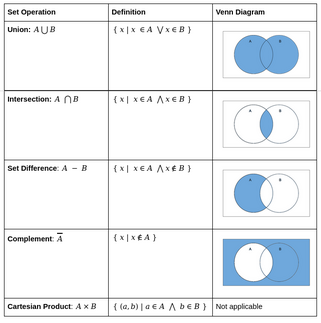### Proving Equivalence of Sets

We can prove two sets A, B are equivalent using the set equivalence laws or by proving A, B are subsets of each other.

Recall $A \equiv B \iff A \subseteq B \land B \subseteq A$

By definition of AB, we can show ABby showing for all objects x, if xA, then xB. So our proofs look as follows:

1. Announce we will prove $A \equiv B$ by showing $A \subseteq B$ and $B \subseteq A$.
2. Show $A \subseteq B$ by showing if $x \in A$, then $x \in B$
3. Then show $B \subseteq A$ by showing if $x \in B$ then $x \in A$.
4. Conclude we have indeed shown $A \equiv B$.

# Module 4 - Set Cardinality

## Lesson 1 - Counting Unions

### Counting Sets

$nucampus = {London, Portland, Boston}$

 Set cardinality - $nucampus$
• The number of elements

GV = {cucumber, kale, asparagus, peas}

Berries = {Black, straw, raspberry, blue berry}

 $GV \cup Berries$= {cucumber, kale, asparagus, peas, black, straw, rasp, blue} = 8
 $GV \cup B = GV + B$
• Is this always true? NO!
• It’s not if you have overlap, because you would count the overlap twice

Remove duplicates:

## Inclusion/Exclusion Principle

### 2 Sets General Form:

 $A \cup B = A + B - A \cap B$

• You have to once more get rid of the overlap (which could be counted an additional time than with 2 sets)

### 3 Sets General Form:

 $A \cup B \cup C = A + B + C - A \cap B - A \cap C - B \cap C + A \cap B \cap C$
• You need to add the shared elements of all 3 again (you cut it out too many times)

### 4 Sets General Form:

 $A \cup B \cup C \cup D = A + B + C + D - A \cap B - A \cap C - B \cap D - C \cap D + A \cap B \cap C + A \cap B \cap D + A \cap C \cap D + B \cap C \cap D - A \cap B \cap C \cap D$

## Lesson 2: Counting Cartesian Products

WestCoast = {Vancoover, Seattle, San Fran, Fan Jose}

EastCoast = {Portland, Charlotte, Boston}

LT = {London, Toronto}

Need one person from each region

FwC = {Bethany, Ian, Jodi}

FeC = {Lany, Maria}

FLT = {Nate, Paul, Samantha}

1 Person to join from each region

### How Many Different Combinations?

FwC x FeC x FLT = {(Bethany, Laney, Nate), (Bethany, Maria, Nate)…}

Let’s visualize this in a tree:How Many Possible 4 Digit Pin Numbers Are There?

Because we multiply how choices we have by the amount of the times we have to make a choice, we get

 Digits = {0,1,2,3,4,5,6,7,8,9} $\therefore$ Digits = 10

10 * 10 * 10 * 10

## Lesson 3: Pigeonhole Principle

Example 1:

7 Teams that are going to have students assigned to

8 Students in the class

What can I say happens when I assign students to a team?

No matter what, one team has at least 2 students

Example 2:

7 Teams

22 Students

No matter the placement/assignment, if all students are on some team then there is 1 team that has at least 4 students

Example 3:

25 Professors

6 TAs

Rule:

• Every professor has exactly 1 TA

What can I say about the number of professors per TA?

Then 1 TA is assigned to 5 professors

### Pigeon Hole Principle - General Definition

If you have k+1 objects in k bins, at least one bin has more than 1 object

### General Formula

If you have n objects to pack into k bins, then at least one bin has at least $\lceil \frac{n}{k} \rceil$ objects

• $\lceil n \rceil$ is a ceiling which means that you round up
• $\lfloor n \rfloor$ is a floor which means that you round down

Example:

25 professors

6 TAs

Every professor has exactly 1 TA

One TA with $\frac{25}{6} = 4 \frac{1}{6}$

$\therefore$ one TA has at least 5 professors if every professor has 1 TA

Example 2:

25 professors

6 TA’s

Rules:

• Every professor has a TA
• No TA has more than 5 professors

At least 3 TA’s are assigned to 4 or more professors

WHY?

If we have 25 professors that need to be assigned to 6 TAs and every professor must be assigned to TA AND no TA is assigned to more than 5 professors then at least 3 TA’s are assigned to 4 or more professors.

A - 25 professors are assigned 6 TA’s and each professor has 1 TA and no TA has more than 5 professors

B - At least 3 TA’s are assigned to 4 or more professors

$A \implies B$

Negation : $A \land \neg B$

Proof by contradiction. Assume not, we have 25 professors assigned to 6 TAs such that every professor is assigned to exactly one TA and no TA has more than 5 professors. We also have by our assumption that fewer than 3 TA’s have 4 or more professors. Then we have at most 2 TAs with 4 or more professors. Consider the case when 2 are assigned 4 and all others are smaller. Then we have 17 professors to assign to 4 TA’s. By the pigeonhole principle we know at least 1 has $\lceil \frac{17}{4}\rceil = \lceil4.25 \rceil = 5$ leaving at least 3 with more than 4. Causing a contradiction $\Rightarrow \Leftarrow$

# Module 5 - Combinatorics and Permutations

## Summary

CS1802 - Counting Handout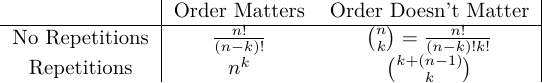## Recitation Notes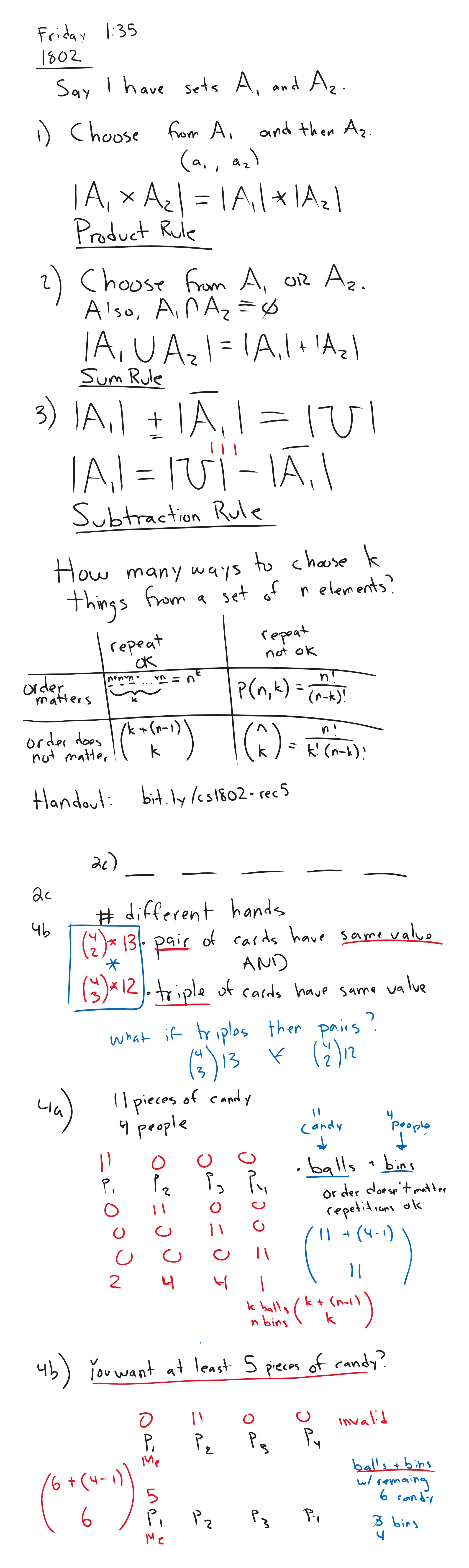## Lesson 1: Permutations (order matters and no repetitions)

How many ways can we order n objects?

A = {a,b,c}

Ex.

• abc
• acb
• bac
• bca
• cba
• cab

6 Ways!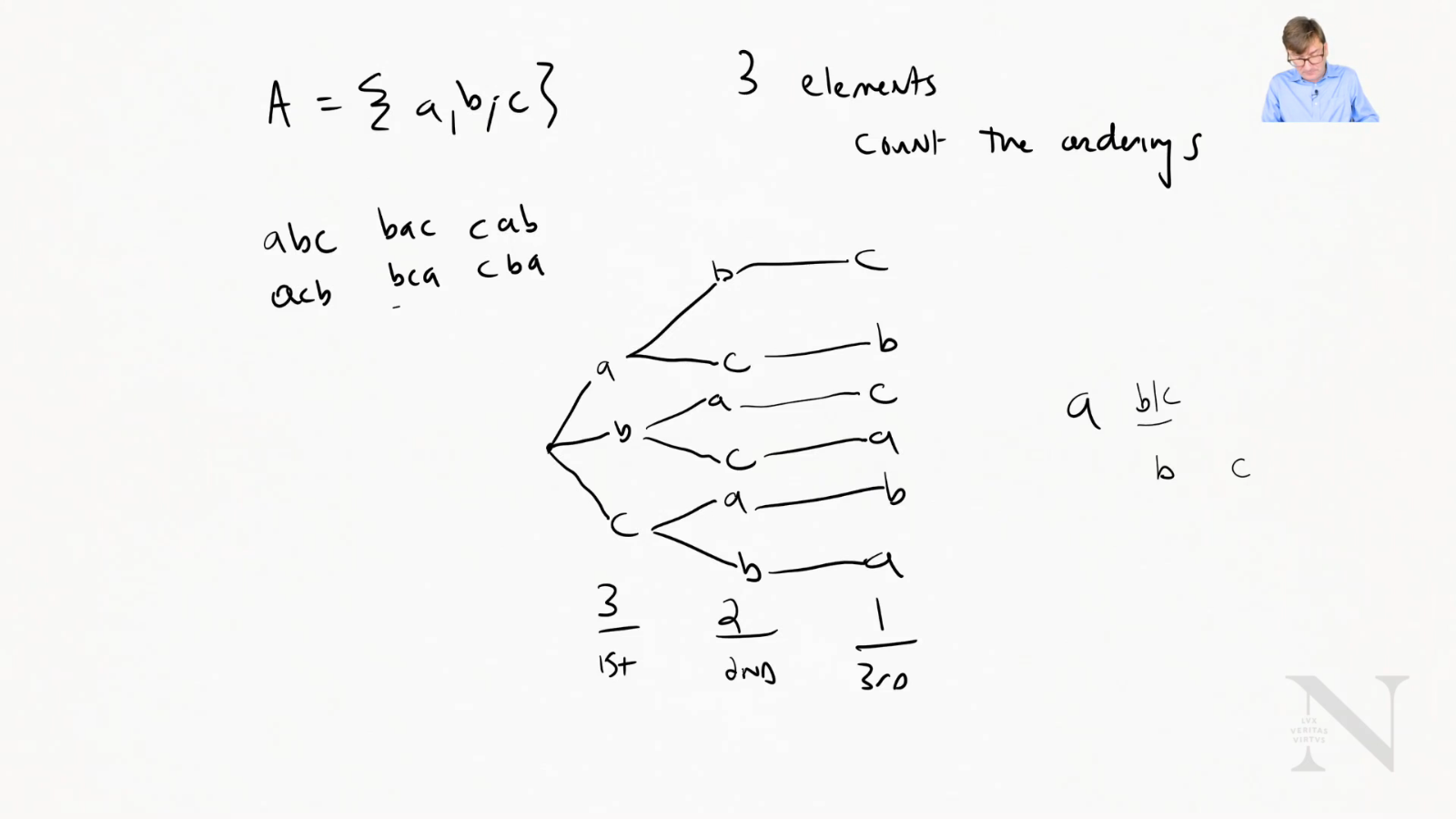### General Formula

3 elements - 321

4 elements - 432*1

$\therefore n!$

Sidenote: $\sum$ for multiplication is $\prod$ therefore $n! =\prod_{i=n}^{1} i$

### Example

How can we choose k elements from a set of n when order matters?

D = {0,1,2,3,4,5,6,7,8,9}

 D = 10

Pick 4 elements

• Order matters
• No repetition

1st choice : 10 possibilities

2nd choice : 9 possibilities

3rd choice : 8 possibilities

4 choice : 7 possibilities

1098*7

Remember: We stopped at k, so it’s no entire factorial. We need to cut off the rest of it

$\frac{n!}{(n-k)!}$ or $p(n,k)$

## Lesson 2: Combinations (Order doesn’t matter and no repetition)

No repetitions

• The default with sets! Assume this!

ex. Choose 2 elements from a set of 4

A = {a,b,c,d}

Recall: order matters would be $\frac{4!}{(4-2)!} = 12$

However $ab=ba$. In this example, that would mean that we cut the options in half

Written as ${4}\choose{2}$ or $c(4,2)$

• Pronounced “4 choose 2”

${n}\choose{k}$$= \frac{n!}{(n-k)!k!} k! is added because we overshot by how many times we can order k elements ### Examples Let’s consider we have 9 people, we want to know the number of possible teams we can make of size 5. {9}\choose{5}$$= \frac{9!}{(9-5)!5!}$

Variant:

9 people, teams of size 5. But 2 people, Logan and Andrew must together if picked.

Two cases

• Logan and Andrew are picked
• ${7 \choose 3}$
• No Logan + No Andrew
• ${7 \choose 5}$

${7 \choose 3} + {7 \choose 5} =$

Variant:

9 people, need team of 5, but Ben can’t work with Nate.

3 Cases

• Ben is on + Nate is out
• ${7 \choose 4}$
• Nate is on + Ben is out
• ${7 \choose 4}$
• Ben is out + Nate is out
• ${7 \choose 5}$

${7 \choose 4} + {7 \choose 4} + {7 \choose 5} =$

Logical Evaluation:

$\neg Ben \lor \neg Nate$

Negate: $Ben \land Nate$

Things that can’t happen:

• Both Ben and Nate are on
• ${7 \choose 3}$

### Difference Method

${9 \choose 5} - {7 \choose 3} = 91$

### Teams with Types

12 People

• 5 Faculty
• 7 Students

Teams of size 5

How many teams have 3 faculty members and 2 students?

${5 \choose 3} \cdot {7 \choose 2} = 210$

How many teams of size 5 contain at most 1 faculty?

2 Cases

• 0 faculty + 5 students
• ${7 \choose 5} \cdot {5 \choose 0}$
• 1 faculty + 4 students
• ${7 \choose 4} \cdot {5 \choose 1}$

${7 \choose 5} \cdot {5 \choose 0} + {7 \choose 4} \cdot {5 \choose 1}$

How many teams have at least 1 faculty members?

5 cases

• 1 fac + 4 students
• ${5 \choose 1} \cdot {7 \choose 4}$
• 2 fac + 3 students
• ${5 \choose 2} \cdot {7 \choose 3}$
• 3 fac + 2 students
• ${5 \choose 3} \cdot {7 \choose 2}$
• 4 fac + 1 student
• ${5 \choose 4} \cdot {7 \choose 1}$
• 5 fac + 0 students
• ${5 \choose 5} \cdot {7 \choose 0}$

${5 \choose 1} \cdot {7 \choose 4} + {5 \choose 2} \cdot {7 \choose 3} + {5 \choose 3} \cdot {7 \choose 2} + {5 \choose 4} \cdot {7 \choose 1} + {5 \choose 5} \cdot {7 \choose 0}$

## Lesson 3: Counting with Repetitions when Order Matters

ex. 4 Digit pin

Digits = {0,1,2,3,4,5,6,7,8,9}

1st choice - 10 options

2st choice - 10 options

3st choice - 10 options

4st choice - 10 options

101010*10

$n^k$

## Lesson 4: Balls and Bins: Counting With Repetitions When Order Doesn’t Matter

ex. Ordering pizza

4 pizza choices

• Cheese
• Pepperoni
• Veggie
• Meat Lovers

How many ways from this set can I get 3 pizzas?

• Create ‘bins’ with dividers
•  ex P C M V (‘1’ denotes a divider)
• If you have a ‘ball’ in a bin, denote it with a 0 and the dividers are 1’s
• ex. 2 pepperonis, 1 cheese = 001011 (looks like binary)

• With 4 categories, you need 3 bars (which means 3 1’s)
• 3 pizzas to pick (which means 3 0’s)

How many binary numbers have 3 0’s and 3 1’s (6 bit)

• 6 bits long and you need to pick a location for the 3 1’s
• ${6 \choose 3}$

ex 2. 10 pizzas, 4 types

• 13 bits
• 3 ‘bars’ (1’s)
• 10 items (0’s)

${13 \choose 3}$

Or we could also ask where the 10 0’s are which would be

${13 \choose 10}$ (it’s the same answer)

### General Formula

n - categories (n-1 1’s)

k - things to pick (k 0’s)

$(k+(n-1)) \choose k$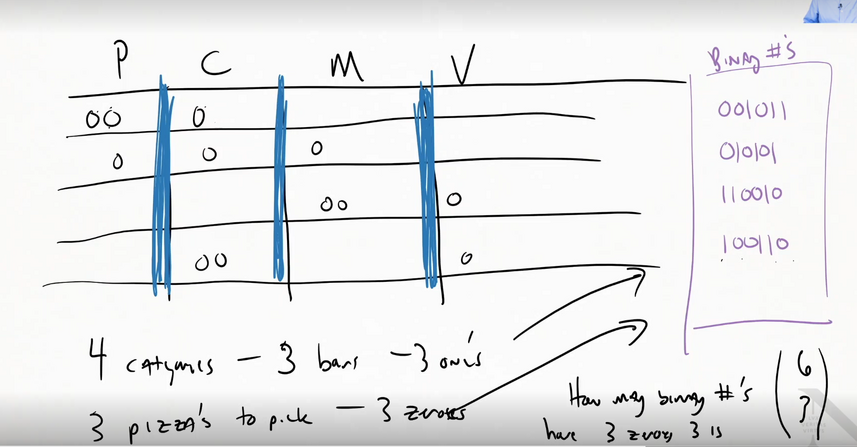# Module 6 - Probability

## Summary

CS1802 - Probability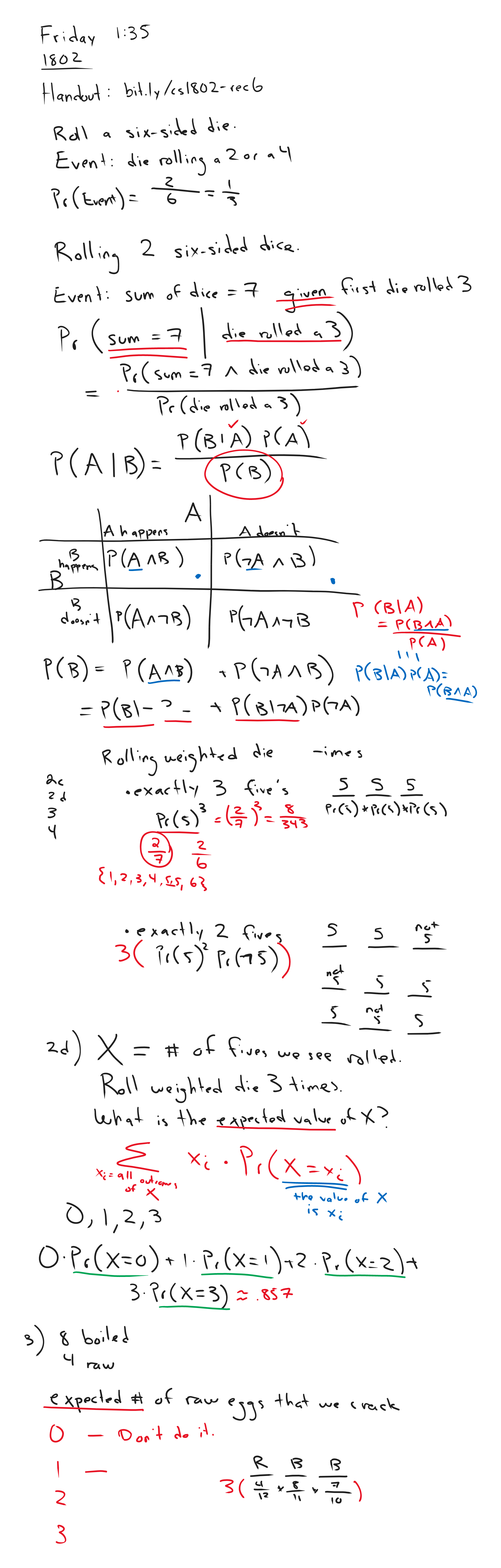## Lesson 1: Overview of Probability

Represents uncertainty

• Makes guesses

## Lesson 2 Part 1: Events, Experiments, Sample Space and Probabilities

An event is something that might happen

An experiment or observation is a situation with one or more possible outcomes

• ex. flipping a coin (2 outcomes)

A probability is a real number in the range [0,1] (inclusive)

• 0 - Will not happen
• 0.5 - 50/50 chance
• 1 - Guaranteed to happen

$Pr(A)$ - Probability of A

$Pr(heads) = 0.5$ or $Pr(C=H) =0.5$

• Where C is a random variable and H is what we’re interested in

### Dice Example

S = {1,2,3,4,5,6}

 $Pr(event) = \frac{ E }{ S }$

Either 5 or 6:

E = {5,6}

$Pr(\text{roll 5 or 6}) = \frac{1}{3}$

### Coin Example

S = {H,T}

E = {E}

$Pr(heads)=0.5$

### Two Dice Example

S = {(1,1),(1,2)….,(6,6)}

 $S = 6\times6= 36$

What is $pr(\text{doubles})$?

E = {(1,1), (2,2) …}

 $Pr(\text{doubles}) = \frac{ E }{ S } = \frac{1}{6}$

Sum to 6:

E = {(1,5), (2,4) …}

$Pr(\text{roll a 6}) = \frac{5}{36}$

Flipping 4 Coins

 $S = 2^{4} = 16$

 $E = {4 \choose 2} = 6$
• We can use our combinations knowledge here

$Pr(\text{2 heads}) = \frac{3}{8}$

## Lesson 2 Part 2: Fallacies to Avoid

• Two outcomes does NOT mean equal chance

### Inclusion-Exclusion Principle

$Pr(E_{1} \cup E_{2}) = Pr[E_1] + Pr[E_2] - Pr[E_1 \cap E_2]$

## Lesson 2 Part 3: Sampling with and Without Replacement

• Without replacement
• Cannot draw an item again
• With replacement
• Can draw an item again

Example: Urns and balls

20 balls

• 18 are red
• 2 are green

### With Replacement

S = {red1, red2, green1…}

Valid Draw: {r1,r1,g1}

Draw 2 Balls:

 $S \times S \times S = 20\times 20\times 20 = 8,000$

$Pr(\text{0 green balls}) = \frac{18^3}{8,000} = 0.729$

$Pr(\text{3 green balls}) = \frac{2^3}{8,000} = 0.001$

### Without Replacement

Valid Draw: {r1,r2,g1}

Sample Size Space: $C(20,3) = 1140$ ←Outcomes

$Pr(\text{0 green}) = \frac{C(18,3)}{1,140} = 0.716$

### Axons of Probability

• Range [0,1]
• $Pr(A) + Pr(\neg A) = 1$ (union of events)
• $Pr(A\cup B) = Pr(A) + P(B) - P(A\cap B)$

## Lesson 3: Independence and Conditional Probability

Events are independent if and only if $Pr(A\land B) = Pr(A) \times Pr(B)$

Example: At least one double in 3 rolls → Independence

$1-Pr(\text{no doubles on 3 rolls})$

Example:

 $Pr(\text{sum of die1 and die2 is 7 die1 is 6})$
•  NOTE: means “given”

When die1 is a 6: {(6,1),(6,2)…}

• 6 outcomes

When sum is 7 and die1 is a 6: {(6,1)}

• 1 outcome
 $Pr(\text{die1 + die2 = 7 die is a 6}) = \frac{1}{6}$

### General Formulas - Dependence

 $Pr(A B) = \frac{Pr(A\land B)}{Pr(B)}$
 $Pr(A B) = \frac{ (A\cap B) }{ B }$ (holds by definition)

### General Formulas - Independence

If and only if A and B are dependent: $Pr(A\land B) = Pr(A) \times P(B)$

 Independence holds if and only if $P(A B) = Pr(A)$

Example: Critical Bugs in Code

$Pr(\text{Bugs}) = Pr (\text{Bugs}\land\text{SB}) + Pr(\text{Bugs}\land\neg\text{SB})$

 $= Pr(\text{Bugs} \text{SB})\times Pr(\text{SB}) + Pr(\text{Bugs} \neg\text{SB}) \times Pr(\neg\text{SB})$

= 0.12

## Lesson 4 Part 1: Mathematical Expectation

Expected Value

• Value you should get if you average over many trials

The expectation of a random variable (r.v.) X is $E[X] = \sum_x Pr(X=x) \times x$

Example:

D = outcome of a 6-sided die

$E[D] = \frac{1}{6}\times 1 + \frac{1}{6} \times 2 + \frac{1}{6} + 3 … \frac{1}{6} \times 6 = 3.5$

Example:

L = outcome of the lottery with 1 in 10 million chance of winning, $2 million payout$E[L] = 2,000,000 (\frac{1}{10,000,000}) + 0\times\frac{9,999,999}{10,000,000} = $0.20$

### Linearity of Expectation

Given rv (random variables) x, y and z=x+y then

$E[z] = E[x]+E[y]$

Example: Let z = sum of 2 six-sided dice. Let x and y be the first and 2nd rolls

$E[z] = E[x] + E[y] = 3.5 + 3.5 = 7$

Look out for this in exams!

## Lesson 5: Bayes’ Rule

How to combine:

• Prior degree of belief
• Evidence

• A model of how likely it is that each hypothesis would produce the available evidence
 $Pr(\text{hypothesis evidence}) \propto Pr(\text{hypothesis}) \times Pr(\text{evidence hypothesis})$

More precise:

 $Pr(\text{hypothesis evidence}) = \frac{Pr(\text{hypothesis}) \times Pr(\text{evidence hypothesis})}{Pr(\text{evidence})}$
• We, however, don’t need to precise answer when comparing two probabilities

### General Formula

 $Pr(\text{A B}) = \frac{Pr(A) \times Pr(B A)}{Pr(B)}$

Bayes’ Rule follows from the definition conditional probability

Derivation

 $Pr(A\land B) = Pr(A B) \times Pr(B) = Pr(B A) \times Pr(A)$
 $Pr(A B) = \frac{Pr(B A) \times Pr(A)}{Pr(B)}$

Example: If I have a bag with 6-sided die and 20-sided die and I pull one out at random and roll a 6. Is it more likely to be a 6 or 20 sided die?

 $Pr(\text{6 6-sided-die}) = \frac{1}{6}$
 $Pr(\text{6 20-sided-die}) = \frac{1}{20}$
 $Pr(\text{6-sided-die 6}) \propto Pr(\text{6 6-sided}) \times Pr(\text{6-sided}) = \frac{1}{12}$
 $Pr(\text{20-sided-die 6}) \propto Pr(\text{6 20-sided}) \times Pr(\text{20-sided}) = \frac{1}{40}$

It is more likely for it to be a 6-sided die

Modify the prior:

• Bag contains 4 20-sided and 2 6-sided

$Pr(\text{6-sided}) = \frac{1}{3}$

$Pr(\text{20-sided}) = \frac{2}{3}$

 $Pr(\text{6-sided-die 6}) \propto Pr(\text{6 6-sided}) \times Pr(\text{6-sided}) = \frac{1}{18}$
 $Pr(\text{20-sided-die 6}) \propto Pr(\text{6 20-sided}) \times Pr(\text{20-sided}) = \frac{1}{30}$

Still more likely for it to be a 6-sided die

## Lesson 6: Variance

Variance: How spread out a distribution is. How far are the samples to the mean on average?

$E[x-E[x]] = 0$ (because the negatives and positives negate each other by definition)

Definition: For a r.v. X, $var(x) = E[(x-E[x]^2)]$ or $var(x) = E[x^2] - E[x]^2$

The standard deviation of x is $\sqrt{var(x)}$

# Module 7 - Sequences, Series, and Induction

CS1802 - Sequences and Series

## Lesson 1: Sequences

Order matters

ex. 3, 10, 17, 24, 31, 38

• Each element is called a term

### Notation

• Counting starts at 1
• $a_1 = \text{1st term} =3$
• $a_2 = \text{2nd term} =10$
• etc.

### Sequence

• A function between a subset natural numbers to a set of numbers
• 3, 10, 17, 24, 31, 38, … (the … means that it goes on forever)
• Notice in this example, there is 7 between each number

### Arithmetic Sequence

• Grows by a fixed amount

Getting an Arbitrary Term:

ex. 3, 10, 17, 24, 31, 38, …

• Start at 3 and add 7 (x-1) times
• 3 + 7 (x-1)
• ex. get the 23rd term: 3 + (23-1) * 7

General Form:

$t(n) = a_1 + (n-1)d$

• $a_1$ is the starting term
• $d$ is the growth factor

### Geometric Sequence

1, 5, 25, 125, 625, 3125, …

• You multiply by 5 each time

$t(n) = 1 \times5^{n-1}$

General Form:

$t(n) = a_1\times r^{n-1}$

• $a_1$ is the first term
• $r$ is the multiplicative factor

ex. 13, 23, 37, 55, 77

• 23-13 = 10
• 37-23 = 14
• 55-37 = 18
• 77-55 = 22

But what if we look at the differences as a sequence?

10, 14, 18, 22, …

• Has a growth factor of 4

In this case, 4 would be the second difference

Quadratic Sequence: **when the 2nd difference is a constant

General Form:

$t(n) = an^{2} + bn + c$

• Recall: this is the quadratic formula

ex. 13, 23, 37, 55, 77

What is the nth term for this?

$t(1) = 13 = a+b+c$

$t(2) = 23 = a*2^{2} + 2b + c = 4a+2b+c$

$t(3) = 37 = a3^2 + b3 +c = 9a+3b+c$

### System of Linear Equations

• System of Linear Equations

13, 23, 37, 55, 77

a+b+c = 13

4a + 2b + c = 23

9a + 3b + c = 37

    9a + 3b + c = 37

+ -4a - 2b - c = -23


— ————————

     5a + b = 14

4a + 2b + c = 23

+  -a - b - c = -13


— ————————

      3a + b = 10

5a + b = 14

+ -3a - b = -10


— —————-

     a = 2


Plug in values and you get:

$t(n) = 2n^2 + 4n +7$

## Lesson 2: Series

Summing up terms:

$\sum_{i=1}^{5} a_i$ ←Sum up the first 5 terms

### General Form:

$\sum_{i=1}^{n} a_i$

Ex.

1+2+3+4+5 = s

• 5+4+3+2+1 = s

— ———————

 6+6+6+6+6 = 2s


### General Form: Arithmetic Series

$\sum_{i=1}^{n} a_i = \frac{(n+1)n}{2}$

$\sum_{i=1}^{n} a_i = \frac{(a+(a+(n-1)d))n}{2}$

### General Form: Geometric Series

Example: $3+\frac{3}{2}+\frac{3}{4}+\frac{3}{8}$

• r = $\frac{1}{2}$
• $n_1 = 3$

$\frac{s}{2} = \frac{3}{2} + \frac{3}{4} + \frac{3}{8} + \frac{3}{16}$

• If you subtract this by $3+\frac{3}{2} + \frac{3}{4} + \frac{3}{8}$ , it results in just the first and last term

$s-r*s = 1-r^n$

$s(1-r) = 1-r^n$

$\therefore s = \frac{a-r^n}{ar}$

## Lesson 3: Proof By Induction

Example: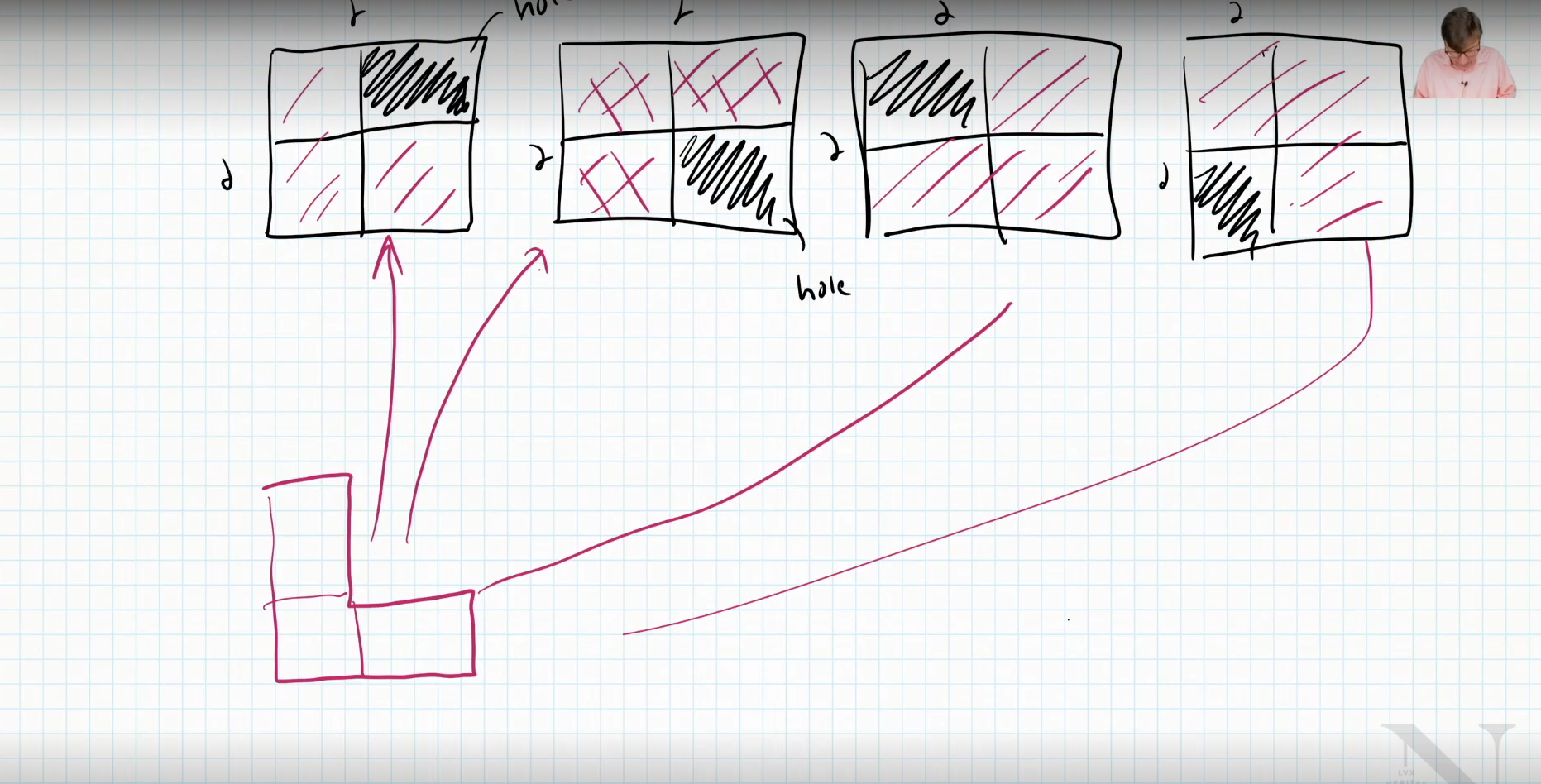We proves that we can fit the L shaped piece into any 2x2 grid

• Yes, because we can split it up into 4 2x2 grids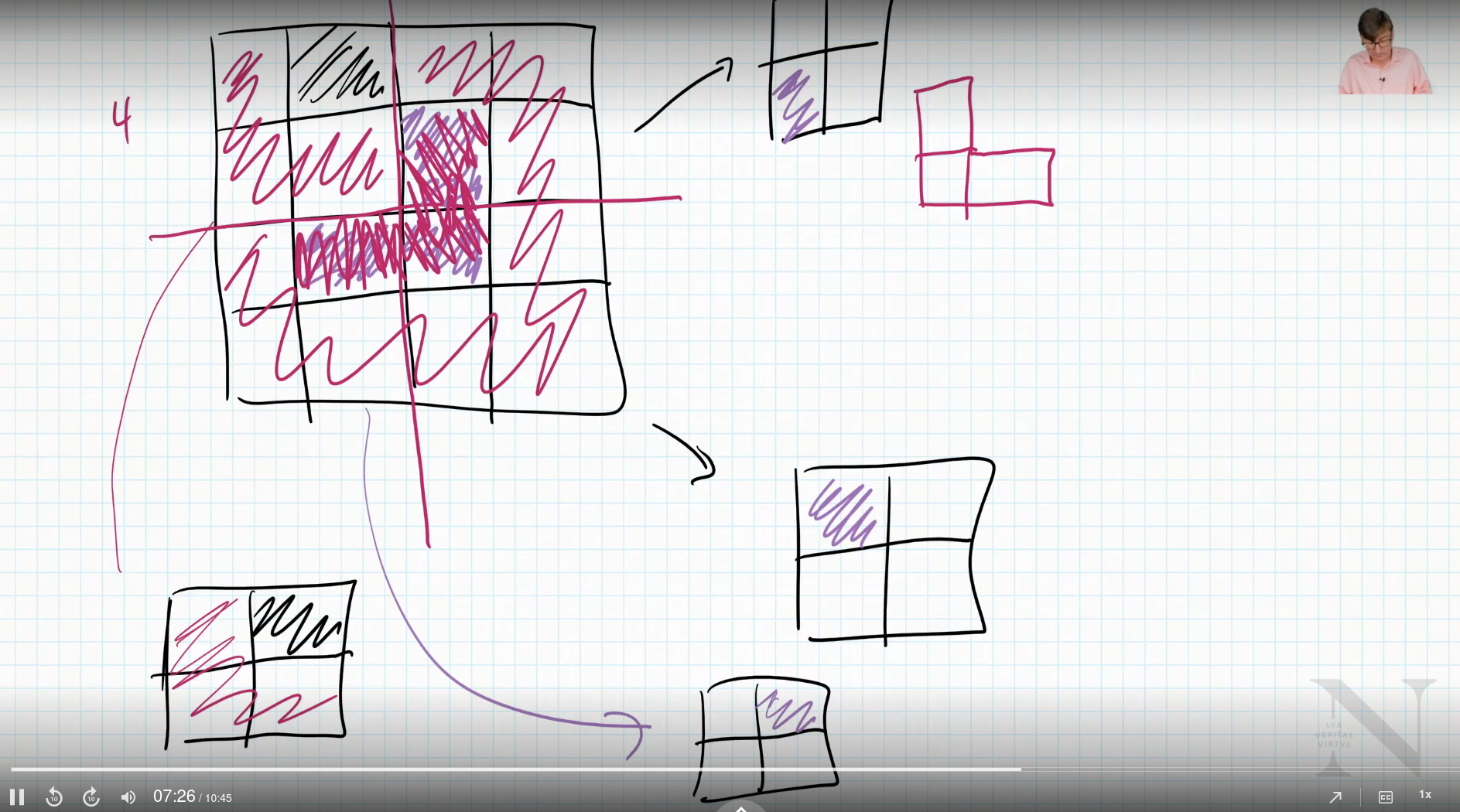• Yes, because we can divide it into 4 4x4 grids

We are essentially building up a foundation

Example 2:

3 cent coin

5 cent coin

• Can we make any amount of change greater or equal to 8 cents?

Assume we have figured out how to make k cents worth of change using 3 cent and 5 cent coins. Can we make k+1 cents?

Case 1:

• No 5 cent coins
• In this case, take 3 3 cent coins away and add 5 cents
• k→k+1

Case 2:

• At least 1 5 cent coin
• In this case, remove 5 cent coin and add 2 3 cent coins
• k→ k+1

Proof by induction

### Definition:

$P$ is some property defined over the integers and $a$ is some fixed integer and

1. $P(a)$ is true (base case)
2. $\forall k\in \mathbb{Z}, n \leq a, P(N)$ (inductive step)

This applies $\forall n \in \mathbb{Z} , n \geq a, P(n)$

Example:

• $P(n)$ - n cents can be made with 3 cent coins and 5 cent coins, $n\geq 8$

Proof by Induction

Consider the base case when n=8, we know that 8 can be made with 1 3 cent coin and 1 5 cent coin. Now consider the inductive hypothesis for an arbitrary k, k≥8. We can make k cents using 3 cent and 5 cent coins. Consider two cases. Case 1 in our k cents. The number of 5 cent coins is zero. Then remove 3 3 cent coins to make k-9 cents. Now add 2 5 cent coins to make k+1 coins. Case 2. We have at least 1 5 cent coin. Remove it to make k-5 cents. Now add two 3 cent coins to make k+1 cents. Since we know P(8) to be true and P(k) implies P(K+1) then P(n) is true for all $n\in \mathbb{Z}, n\geq 8$. Q.E.D.

Example:

$\sum_{i=1}^{n} i = 1+2+3…+n = \frac{n(n+1)}{n}$

Prove this by induction

• Base case
• Inductive step

Proof by Induction

Consider the base case when n=1 then we know $\frac{1(1+1)}{1} = 1$ which is the series with one term. Let’s assume for some $k$ $1+2+3…k = \frac{k(k+1)}{2}$ by our inductive hypothesis. Consider $1+2+3 …+k+(k+1)$which is $\frac{k(k+1)}{2} + (k+1)$ by our inductive hypothesis.

$\frac{k(k+1)}{2} = \frac{(k+2)(k+1)}{2}$

So we have $1+2+3…+k+(k+1) = \frac{(k+2)(k+1)}{2}$ as desired. So it is true for all n. Q.E.D.

## Lesson 4: Strong Induction

Strong Mathematical Induction

$P(n)$ property defined over the integers with a, b fixed integers, a≤b. Base P(a), P(a+1), P(a+2), … P(b) are all true. Inductive $\forall k \geq b$ if P(i) is true $\forall i, a\leq i\leq k$ then P(k+1) is true. This implies $\forall n, n\geq a, P(n)$ is true.

Consider the Sequence:

$a_0 = 0,\ a_1=4,\ a_k = 6 \times a_{k-1} - 5 \times a_{k-2}$

$\therefore a_{2} = 6\times 4 - 5 \times 0 = 24$

$a_3 = 6\times 24 - 5 \times 4 = 124$

Claim $a_n = 5^n - 1$

• This needs strong induction

$P(n) = a_k = 6\times a_{k-1} - 5 \times a_{k-2}$

$a_n = 5^n -1$

### Proof by Strong Induction

Consider the base case $P(0),\ P(1),\ P(2)$

$P(0) = a_0=0=5^0 -1 = 1-1 =0$

$P(1) = 4 = 5^1-1$

$P(2) = 24 = 5^2 - 1$

Consider the case when n is 0, we know that $5^0-1 = 0$, which is $a_0$ as defined. We know $a_{k+1}$is $6\times a_k - 5\times a_{k+1}$ by definition. Inductive hypothesis is that P(i) is true for all 0≤i≤k, $a_i = 5^1 -1.$ Consider $a_{k+1} = 6\times(a_k)-5 \times a_{k-1}$ which is $6(5^k -1) - 5(5^{k-1}-1)$ (string induction). Therefore $a_{k+1} = 5^{k+1} -1$. $\forall n, a_n = 5^n -1$. Q.E.D.

## Lesson 5: Recurrences

Looking at recursion.

Substitution Method:

Ex.

T(0) = 5 (base case)

T(n) = T(n-1) + n (next step)

How can we define T(n) without T?

• Recurrence relation

T(0) = 5

T(n) = T(n-1) + n

T(1) = T(0) + 1

T(3) = T(1) + 2 = T(0) 1 + 2

T(4) = T(2) + 3 = T(0) 1 + 2 + 3

T(5) = T(3) + 4 = T(0) 1 + 2 + 3 + 4

T(n) = T(0) + 1 + 2 + 3 …

$T(n) = T(0) + \frac{n(n+1)}{2} = 5 + \frac{n(n+1)}{2}$

Example 2:

T(1) = 1

T(n) = n + 2 * T(n/2)

$T(\frac{n}{2}) = \frac{n}{2} + 2\times T(\frac{n}{4})$

$T(n) = n+ 2( \frac{n}{2} + 2\times T(\frac{n}{4}))$

$T(\frac{n}{4}) = \frac{n}{4}+ 2\times T(\frac{n}{8})$

$T(\frac{n}{8}) = \frac{n}{8} + 2\times T(\frac{n}{16})$

$T(n) = n+2(\frac{n}{2} + 2(\frac{n}{4} + 2(\frac{n}{8} + 2\times T(\frac{n}{16}))))$

$T(n) = n+n+n+… 2^k\times T(\frac{n}{k})$

$T(n) = kn +2^k\times T(\frac{n}{k})$

Goal:

• No T’s on the right hand side

$T(1) = 1$ so what value of k does $T(\frac{n}{2^k}) = T(1)$

$\frac{n}{2^k} = 1$

$n = 2^k$

$\therefore k = log_2(n)$

$T(n) = n\times log_2 (n) + n$

# Module 8 - Growth of Functions with Sorting

## Lesson 1: Sorting a List of Number: Insertion Sort

We want to ask: How good are these sorting algorithms?

Input: 7,3,2,4 Output: 2,3,4,7

A list with one element is always sorted

This is an invariant which means that it is always true (never varies).

### Insertion Sort:

1. Swap until it is in the correct location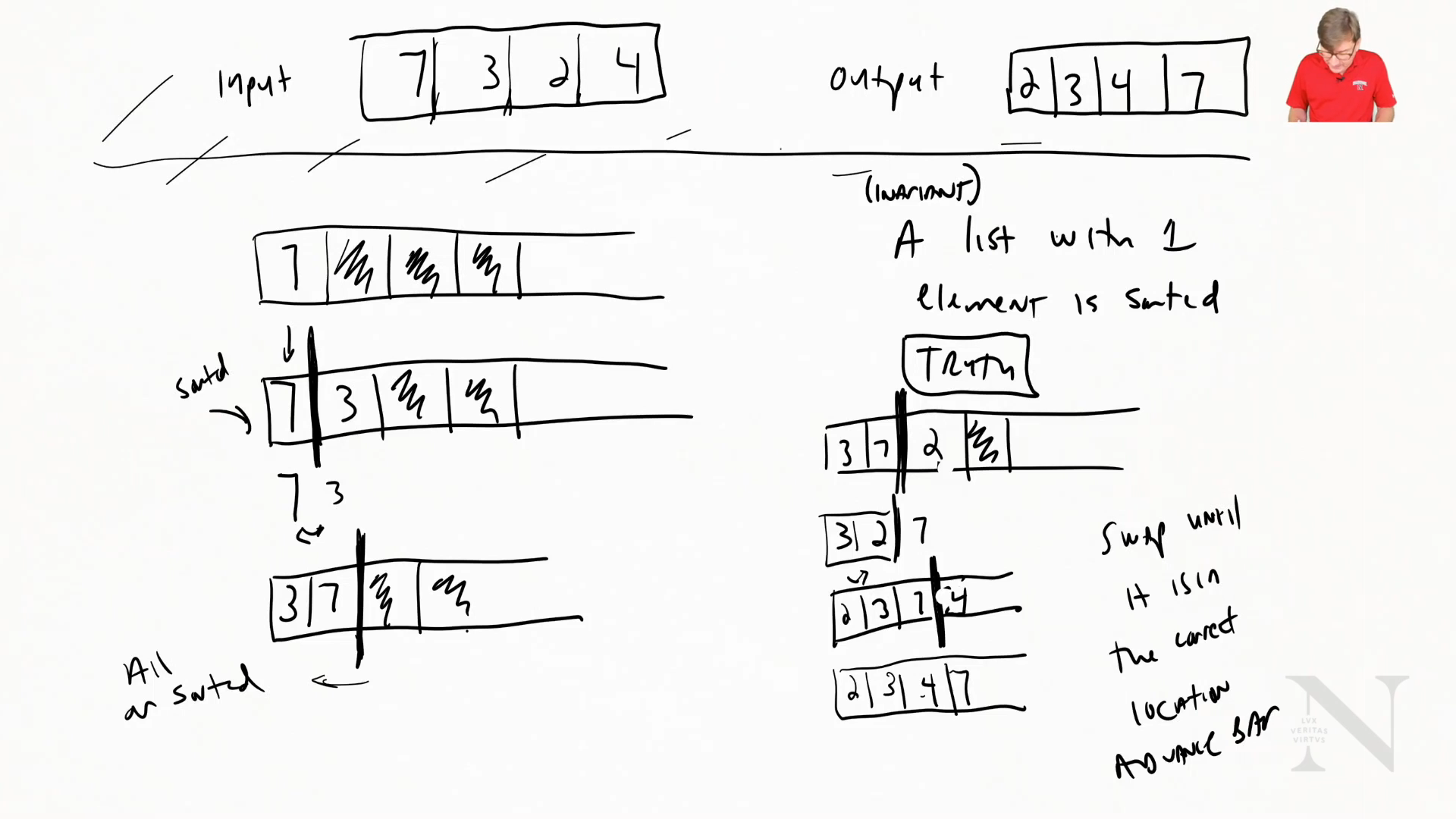insertionSort(A)
for i from 1 to n-1:
current := A[i] // Current is one to insert
j := i-1 // Counter to end
while j>= 0 and A[j] > current:
swap(A[j], current)
j=j-1


How expensive is this?

Loop through every element -1

What doe sit do? Possible operations?

• Assignment
• Index into an array
• Subtraction
• Comparison

(These are called atomic operations)

A swap is 3 assignments

Atomic operations cost 1

• Any one thing that a computer does

We need to count the loop

• Worse case scenerio

for loop: n-1 iterations

while loop

• i=1 only 1 time
• i=2 most=2
• i=3 most=3

$\therefore$ Worst case: $\frac{(n-1)(n)}{2}$

## Lesson 2: Sorting a List of Numbers: Merge Sort

Recall: a list of size 1 is already sorted

Merge Sort

• Take two lists and compare first element and remove the smaller one

Does long does this take?

• Worst case scenerio? (in terms of n)

2n comparisons to merge sorte lists

What we know:

• List of size 1 is sorted
• Merging list is “fast”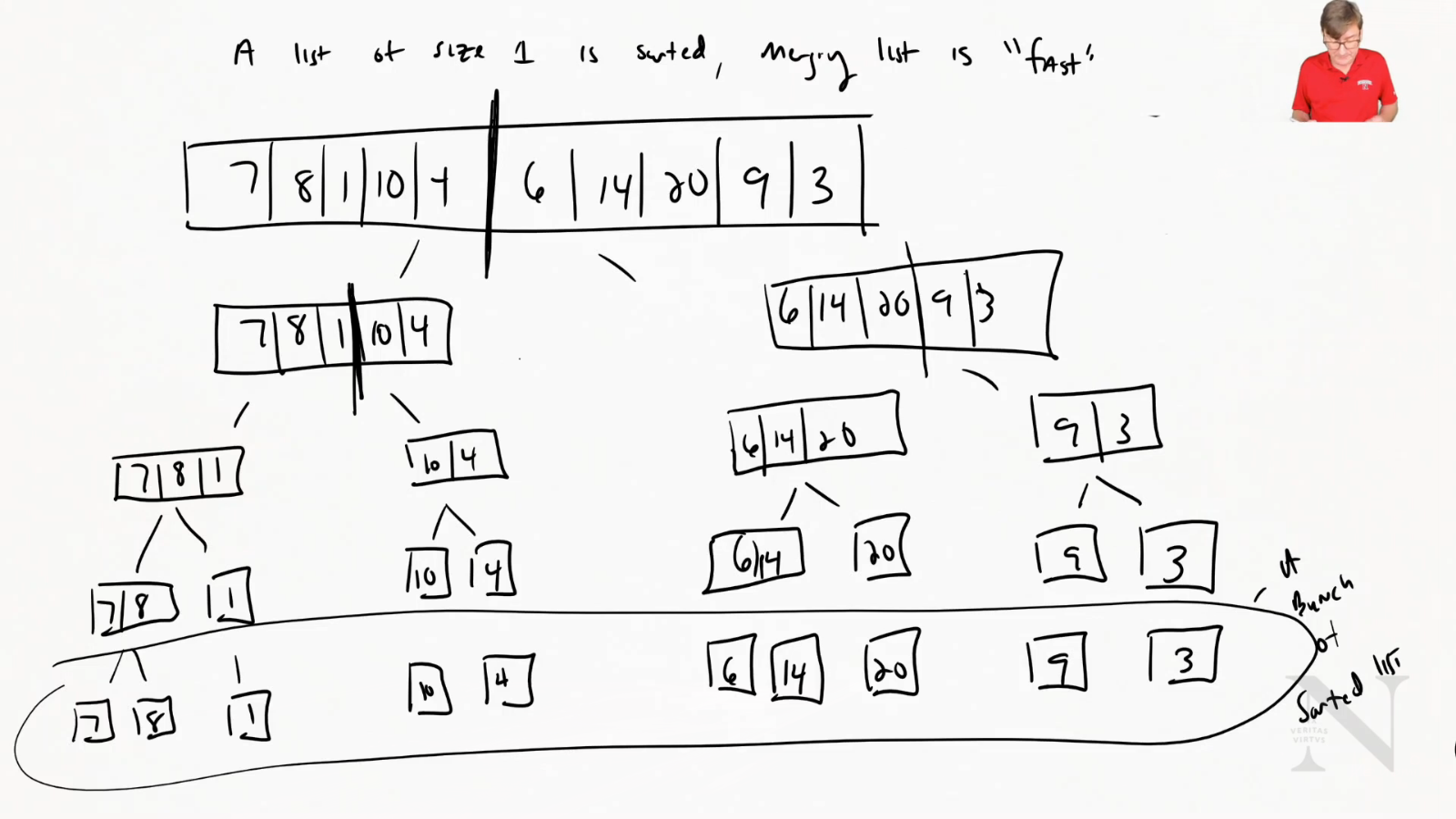Now we can merge them quickly

Number of ways to have a number n is lg(n)

$\therefore \text{cost} = n\cdot lg(n)$

Code:

mergeSort(A, left, right)
if |A| > 1
middle = left + (right-left)/2
B = mergeSort(A, left, middle)
C = mergeSort(A, middle+1, right)
merge(B, C)

merge(A, B)
c = []
while (|A| > 0 and |B| > 0)
if (A < B)
remove A add to back of c
else
remove B add to back of c


Is merge sort better than insertion sort? Yes!!

$\text{Merge Sort}: \approx n\cdot lg(n)$

$\text{Insertion Sort}: \frac{n(n-1)}{2} \approx n^2$

IMAGE:

![[Module 8 - Growth of Functions with Sorting/Screenshot_from_2020-12-01_21-04-43.png]]

## Lesson 3: Asymptotic and Big O

We want to make algorithms efficent

We’re going ot focus on steps

• What is the growth rate of the change

Consider this code:

someFunc(A) //where |A| = n
n = |N| // Cost 2
while (n>0) //Cost 1
{
temp = x // Cost 2
x = y // Cost 1
y = temp // Cost 1
n = n - 1 // Cost 2
}


The total cost of this code is 7n+3

How different is 7n+3 and n when comparing function order?

• The gap is insignificant when we go to infinity

### Big O Notiation

Classify function (group them) so we can compare them.

Let f(n) and g(n) be function mapping non-negative integers to real numbers.

f(n) is O(g(n)) if there exists a real constant c>0 and an integer $n_o >= 1$ such that $f(n) <= c\cdot g(n) \forall n >= n_o$

Claim: 7n+3 is O(n)

• They are the same class

Proof

Let c=8, then we konw that $7n+3<=8n$ for all $n>=3$. By the definition of Big O, 7n+3 is o(n).

## Lesson 4: Ordering Functions

Big Omega

F(n) is $\Omega$(g(n)) if there exists a real constant c>0 and integer $n_o >=1$ such that $c\cdot g(n) <= f(n) \forall n >= n_o$

Big Theta

f(n) is $\Theta$(g(n)) if there exists constants $c_1>0,c_2>0\text{and} n_o >=1$ such that $c_1 \cdot g(n) <= f(n) <= c_2 \cdot g(n)$

$f(n) \text{is} O(g(n)) => f(n) <= g(n)$

$f(n) \text{is} \Omega(g(n)) => f(n) >= g(n)$

$f(n) \text{is} \Theta(g(n)) => f(n) = g(n)$

Little

f(n) is o(g(n)) if for all constants c>0, $\exists n_o > 0, f(n) < c\cdot g(n)\forall n >= n_o$

f(n) is $\omega(g(n))$ (little omega) if for all constants c>0, $\exists n_o >0, f(n) < c\cdot g(n) \forall n>= n_o$

f(n) is $\theta(g(n))$ (little theta) if for all constants c>0, $\exists n_o >0, c\cdot g(n) < f(n) \forall n>= n_o$

Size of Functions

In order of size:

constants, logs, polylogorithms, polynomials, exponential

Note: Exponential algorithms are not possible